Home > ACC7 > Chapter cc27 > Lesson cc27.1.3 > Problem7-37

7-37.
1. Simplify each expression. Homework Help ✎

1. 18 ÷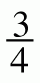2.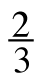(9 − 6) + 40

3. 15 ÷ 5 +· ( −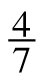)

4.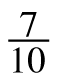+ (−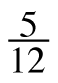) − (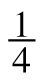)

5. 4.25 – 7.06

6.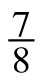− (−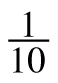) + (−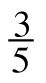)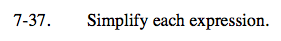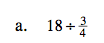Convert 18 to a fraction greater than one, then divide.

24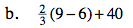Follow the Order of Operations. First simplify any terms in parentheses, then any exponents, then terms being multiplied or divided, and lastly, combine any terms that are being added or subtracted.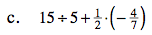See part (b).

$2\frac{5}{7}$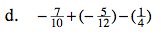Find a common denominator.

$-\frac{164}{120}\text{ or } -1\frac{11}{30}$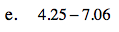See part (b).

−2.81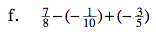See part (d).